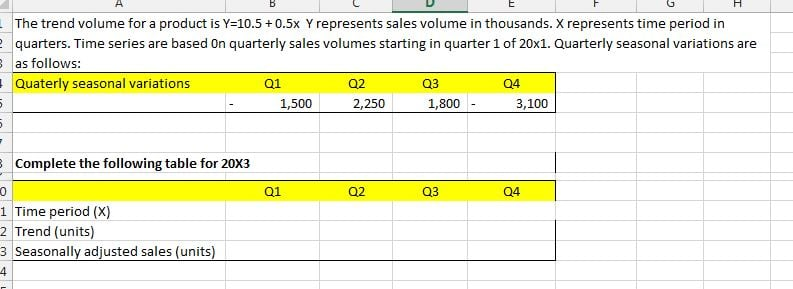# Y = a + bx for calculating seasonally adjusted sales

Hi All,

I came across a calculation which I could not resolve. Perhaps I was not looking from a correct angle. Can someone help me with it? I have attached a picture of the question.• I will start and perhaps someone will add their thoughts on this.

1. I have converted formula to Y = 10,500 + 500x since seasonal variances are in thousands.
2. I have worked out x=9 for 20X3 Q1 (based on 20X1 Q1 = 1)
3. Trend volume for 20X3: Q1 = 15,000, Q2 = 15,500, Q3 = 16,000, Q4 = 16,500
4. No clue afterwards.. What should go to "Time period(x) in Q1 to Q4? I can work out actual data based on trend + variance. But what on earth is this Time period(x)?
• Maybe Time period(x) is 9 for Q1, 10 for Q2, 11 for Q3 and 12 for Q4.
Then Trend (units) Q1=15,000, Q2=15,500, Q3=16,000, Q4=16,500
Then Seasonally adjusted sales Q1=14,000, Q2=17,750, Q3=17,800, Q4=13,400
Perhaps I've just answered my own question...
• Do you know what the answer should be?
• andrew96 said:

Do you know what the answer should be?

Not 100% sure if my assumptions on the latest reply were correct. I do not see any other logical possibility to resolve this.
• Well-Known FarehamRegistered Posts: 247
JJ1 said:

Maybe Time period(x) is 9 for Q1, 10 for Q2, 11 for Q3 and 12 for Q4.
Then Trend (units) Q1=15,000, Q2=15,500, Q3=16,000, Q4=16,500
Then Seasonally adjusted sales Q1=14,000, Q2=17,750, Q3=17,800, Q4=13,400
Perhaps I've just answered my own question...

This looks correct to me.
AAT
Level 2 - 2011
Level 3 - 2012
Level 4 - 2013

ACCA
F4 - Corporate Law - Dec 2015 (passed)
F5 - Performance Management - Dec 2014 (passed)
F6 - Taxation - Dec 2013 (passed)
F7 - Financial Reporting - Jun 2014 (passed)
F8 - Audit & Assurance - Dec 2015 (passed)
F9 - Financial Management - Jun 2015 (passed)